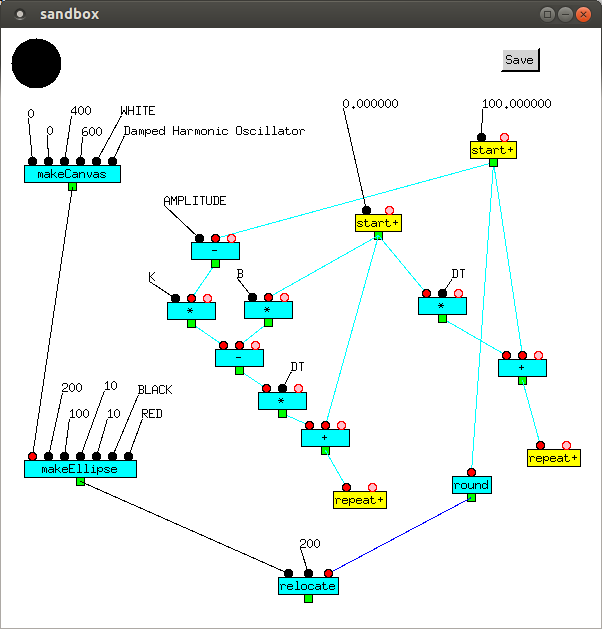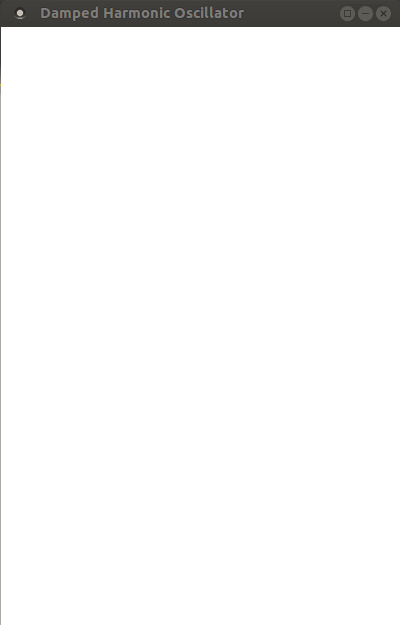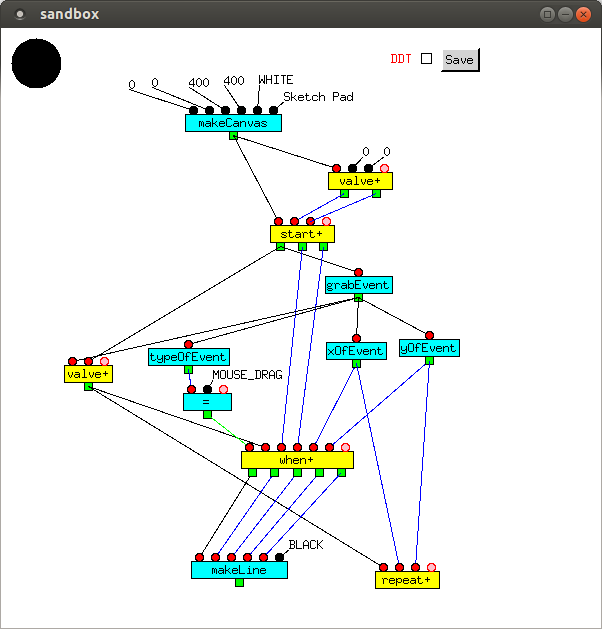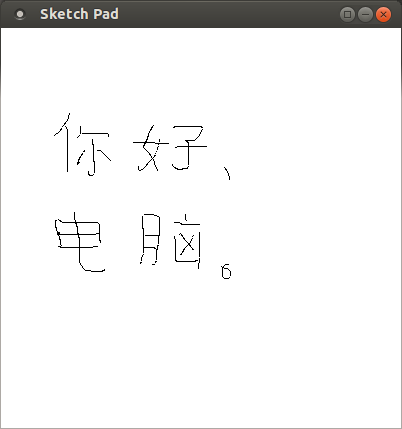# X11 Graphics and Events

X11 (also called Xlib or X Windows), is the 2D graphics and event library available on most Unix systems, and which has been used to develop Full Metal Jacket's development environment.

Here, we use it to develop two simple programs, a damped harmonic oscillator and a sketch pad.

## Damped Harmonic Oscillator

In general, a damped harmonic oscillator has the equations

```  y' = y + v * dt
v' = v + a * dt
where
m * a = spring force + damping force = - k * (y - y0) - b * v
```

The spring force is proportional to the distance from the equilibrium value, and the damping force is proportional to the velocity, opposite in direction to them. The mass oscillates back and forth through the equilibrium point, each oscillation smaller than the last.

In the system modelled below,

```  dt = 0.01
y0 = 300
m = 1
k = b = 0.1
a = - k * (y - y0) - b * v
= - 0.1 * (y - 300) - 0.1 * v
= 0.1 * (300 - y) - 0.1 * v
```
so we have
```  y' = y + v * 0.01
v' = v + a * dt = v + (0.1 * (300 - y) - 0.1 * v) * 0.01
```

It is necessary to have separate loops for y and v. Values of v are updated from their initial value of 0.0 in the master feedback loop (left), and values of y are updated from their initial value of 100.0 in the slave feedback loop (right). To model the system graphically, we have a circle move up and down on a canvas. The canvas, created by `makeCanvas`, is located at (0, 0) on the screen with dimensions 400 x 600. Its color is `WHITE`, and its title is "Damped Harmonic Oscillator". The ellipse, created by `makeEllipse`, is initially at (200, 100), has dimensions 10 x 10 (i.e. is a circle), and is `RED` with a `BLACK` outline. `relocate` changes its position. The other named constants are `K` (0.1), `B` (0.1), `DT` (0.01) and `AMPLITUDE` (300.0).To run, the inputs to the slave loop, the master loop and any input of `makeCanvas` are clicked on in that order.

It is possible to change values of the constants while the system is running, with immediate effect. At present, input is by typing in the new value, but in future, input of "constants" using sliders and other GUI components will be added.

The output is:Keyboard and mouse events can be captured by `grabEvent`, which accepts a `Canvas` as input and returns an `Event`. Events include left, middle, and right button click, button double click, and mouse drag, as well as primitive X11 events such as key press, enter notify, and leave notify.
Here, we implement a simple sketch pad, which allows us to draw on a canvas. If the event is `MOUSE_DRAG`, a line is drawn from the mouse's previous position to its current position. Along with the `Canvas`, the mouse's position, is fed back to `start+` and is used next time as the previous position. All other events are ignored.